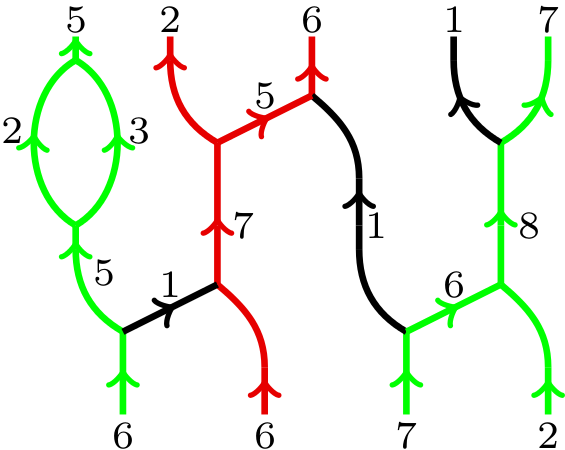## Abstract

We use super q-Howe duality to provide diagrammatic presentations of an idempotented form of the Hecke algebra and of categories of $$\mathfrak{gl}_n$$-modules (and more generally $$\mathfrak{gl}_{N|M}$$-modules) whose objects are tensor generated by exterior and symmetric powers of the vector representations. As an application, we give a representation theoretic explanation and a diagrammatic version of a known symmetry of colored HOMFLY-PT polynomials.

## A few extra words

We discuss a machine that “takes dualities and produces diagrammatic presentations of the related representation theoretical categories”. Specifically, we feed the machine with super q-Howe duality between the superalgebra $$\textbf{U}_q(\mathfrak{gl}(m|n))$$ and $$\textbf{U}_q(\mathfrak{gl}_N)$$.
We construct diagrammatic presentations of an idempotented form of the Iwahori-Hecke algebra as well as of categories of $$\textbf{U}_q(\mathfrak{gl}_N)$$-modules by using the “green-red” web categories $$\infty\text{-}\!\textbf{Web}_{\mathrm{gr}}$$ and $$N\text{-}\!\textbf{Web}_{\mathrm{gr}}$$. Morphisms in these $$\mathbb{C}_q$$-linear categories are combinations of planar, upwards oriented, trivalent graphs with edges labeled by positive integers and colored black, green or red modulo local relations.
An example of a green-red web is:A very similar approach works for the corresponding categories of $$\mathfrak{gl}_{N|M}$$-modules as well as we show in an extra section.